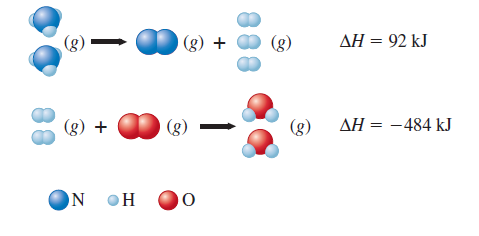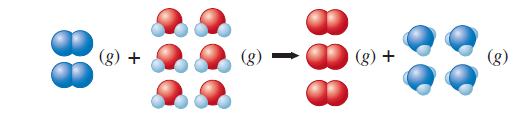# Problem: Given the following data:Calculate ΔH for the reaction On the basis of the enthalpy change, is this a useful reaction for the synthesis of ammonia?

🤓 Based on our data, we think this question is relevant for Professor Pontius' class at PSU.

###### FREE Expert Solution

We start solving this by setting up the equations according to the structures drawn

(1) 2NH3 (g) → N2 (g) + 3H(g)    ΔH°rxn = 92 kJ

(2) 2H2 (g) + O2 (g) → 2H2O (l)    ΔH°rxn = -484 kJ

To find the ΔHrxn of the third reaction, we need to use the Hess's Law where we have to rearrange the given equations to get the desired reaction.###### Problem Details

Given the following data:Calculate ΔH for the reactionOn the basis of the enthalpy change, is this a useful reaction for the synthesis of ammonia?